## Example Questions

### Example Question #9 : Sequences

The sequence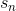is defined by: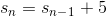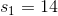What is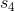?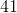Explanation:

Begin by interpreting the general definition:This means that every number in the sequence is five greater than the element preceding it.  For instance: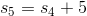It is easiest to count upwards: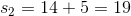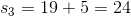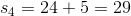### Example Question #10 : Sequences

The sequenceis defined by: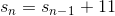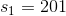What is the value of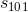?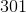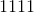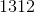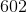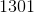Explanation:

For this problem, you definitely do not want to "count upwards" to the full value of the sequence.  Therefore, the best approach is to consider the general pattern that arises from the general definition:This means that for every element in the list, each one isgreater than the one preceding it.  For instance: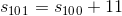Now, notice that the first element is: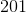The second is: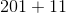The third could be represented as: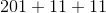And so forth...

Now, notice that for the third element, there are only two instances of.  We could rewrite our sequence: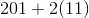This value will always "lag behind" by one.  Therefore, for the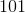st element, you will have: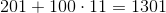### Example Question #11 : Sequences

The sequenceis defined by: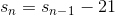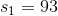What is the value of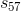?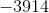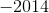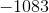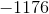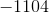Explanation:

For this problem, you definitely do not want to "count upwards" to the full value of the sequence.  Therefore, the best approach is to consider the general pattern that arises from the general definition:This means that for every element in the list, each one isless than the one preceding it.  For instance: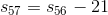Now, notice that the first element is: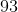The second is: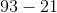The third could be represented as: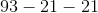And so forth...

Now, notice that for the third element, there are only two instances of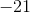.  We could rewrite our sequence: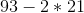This value will always "lag behind" by one.  Therefore, for the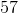th element, you will have: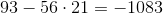Tired of practice problems?

Try live online GRE prep today.